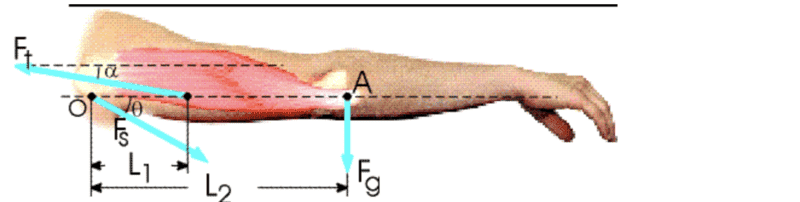# Trying to Figure out Tension force angle on Arm

• Spocktastic
In summary, the conversation discusses solving for three different parts, with a focus on using the law of static equilibrium to determine the necessary x and y components of the forces. The result of part a is a tension force of 870N in the deltoid, while part b involves finding the x and y components for Fs using the deltoid tension force and the weight of the arm. The magnitude of Fs is then calculated using the Pythagorean theorem. For part c, using the x and y components from part b, an attempt is made to find the angle using arctan, but the result is incorrect. The conversation ends with a request for equations and clarification on the directions of the two forces.

#### Spocktastic

Homework Statement
The arm in the figure below weighs 43.2 N. The force of gravity acting on the arm acts through point A. Assume that L1 = 0.0890m, L2 = 0.342m and alpha = 11.0deg.
a) Determine the magnitude of the tension force Ft in the deltoid muscle.
b) Determine the magnitude of the tension force Fs of the shoulder on the humerus (upper-arm bone) to hold the arm in the position shown.
c) Determine the angle of tension force Fs relative to the x-axis.
Relevant Equations
Static Equilibrium: Fnet = 0 & Torque net = 0
a^2 + b^2 = c^2
arctan = Fsin(angle)/Fcos(angle)I already solved for part a, setting the sum of the Torques of the arms and deltoid equal to 0 and subbing in values which lead to a tension force of 870N in the deltoid.

For part b, I remembered the law of static equilibrium, so the summation x and y components of all the forces in the system must equal 0. I found that the x component for Fs needs to be 854.019 by multiplying the deltoid tension force by cos(11) and the y component for Fs to be the sum of the weight of the arm and the deltoid tension force*sin(11), which was 209.2. I used Pythagorean theorum to find that the magnitude of Fs is 879.27N.

Now for part c, I tried using the x and y components I got for part b and substituted them into arctan, so arctan(209.2/854.019) which resulted in me getting 13.76deg. However, it is wrong even when I try to answer it as -13.76. Is it the x-y components I messed up on?

Hello @Spocktastic. Welcome to PF!

Please present your equations. Its better if we can see directly what you did to get your result. This generally makes it easier for anyone helping to review your work.

$$\rightarrow^+ \sum F_x = ...$$

$$\uparrow^+ \sum F_y = ...$$

$$\circlearrowright^+ \sum M_O = ...$$

Please try to format them using Latex, it doesn't take long to learn.

Last edited:
Spocktastic said:
the sum of the weight of the arm and the deltoid tension force*sin(11)
In which directions do those two forces act?